Documentation

## Linearize at Trimmed Operating Point

This example shows how to linearize a model at a trimmed steady-state operating point (equilibrium operating point) using the Linear Analysis Tool.

The operating point is trimmed by specifying constraints on the operating point values, and performing an optimization search that meets these state and input value specifications.

1. Open the Simulink® model.

```sys = 'magball'; open_system(sys)```2. Open the Linear Analysis Tool for the model.

In the Simulink model window, select Analysis > Control Design > Linear Analysis.

3. In the Simulink model window, define the portion of the model to linearize for this linearization task:

1. Right-click the Controller block output signal (input signal to the plant). Select Linear Analysis Points > Input Perturbation.

2. Right-click the Magnetic Ball Plant output signal, and select Linear Analysis Points > Open-loop Output.

Annotations appear in the model indicating which signals are designated as analysis points.

### Tip

Alternatively, if you do not want to introduce changes to the Simulink model, you can specify the analysis points in the Linear Analysis Tool. For more information, see Specify Portion of Model to Linearize in Linear Analysis Tool.

4. Create a new steady-state operating point at which to linearize the model. In the Linear Analysis Tool, in the Operating Point drop-down list, select Trim model.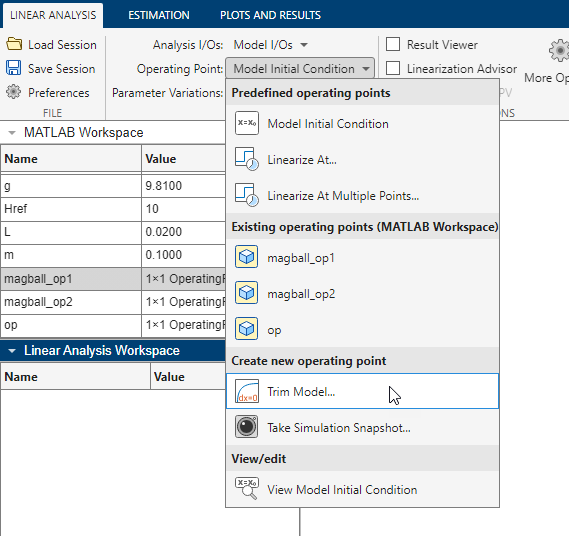In the Trim the model dialog box, the Specifications tab shows the default specifications for model trimming. By default, all model states are specified to be at equilibrium, indicated by the check marks in the Steady State column.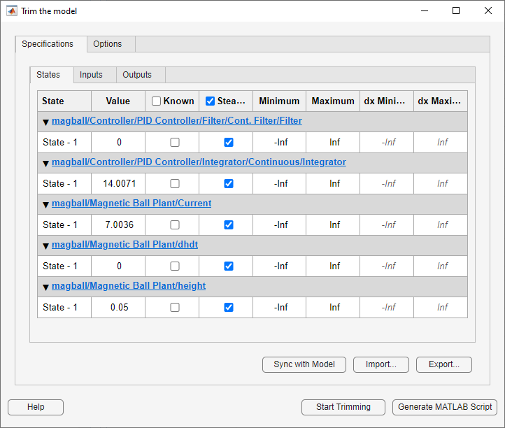5. Specify a steady-state operating point at which the magnetic ball height remains fixed at the reference signal value, 0.05. In the States tab, select Known for the height state. This selection tells Linear Analysis Tool to find an operating point at which this state value is fixed.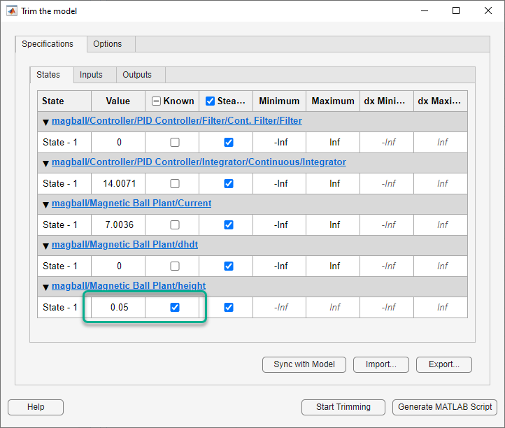6. Since the ball height is greater than zero, the current must also be greater than zero. Enter `0` for the minimum bound of the Current block state.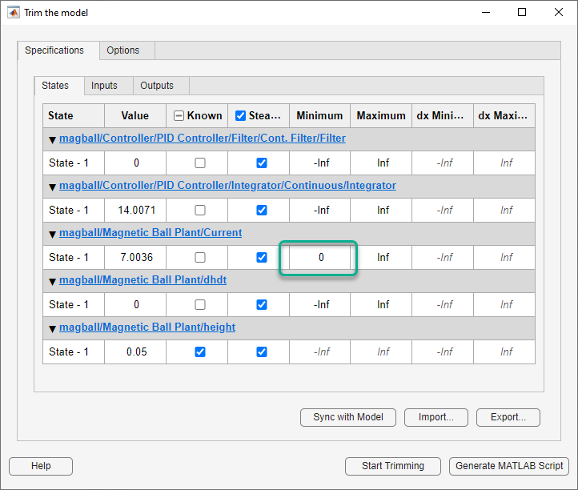7. Compute the operating point.

Click.

A new variable, `op_trim1`, appears in the Linear Analysis Workspace.In the Operating Point drop-down list, this operating point is now selected as the operating point to be used for linearization.

8. Linearize the model at the specified operating point and generate a bode plot of the result. ClickBode. The Bode plot of the linearized plant appears, and the linearized plant `linsys1` appears in the Linear Analysis Workspace.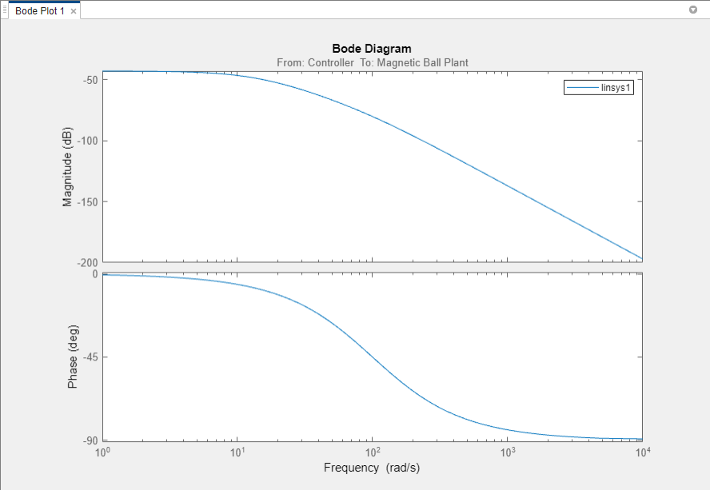### Tip

Instead of a Bode plot, generate other response types by clicking the corresponding button in the plot gallery.

Right-click on the plot and select information from the Characteristics menu to examine characteristics of the linearized response.

## Related Topics

##### SupportGet trial now